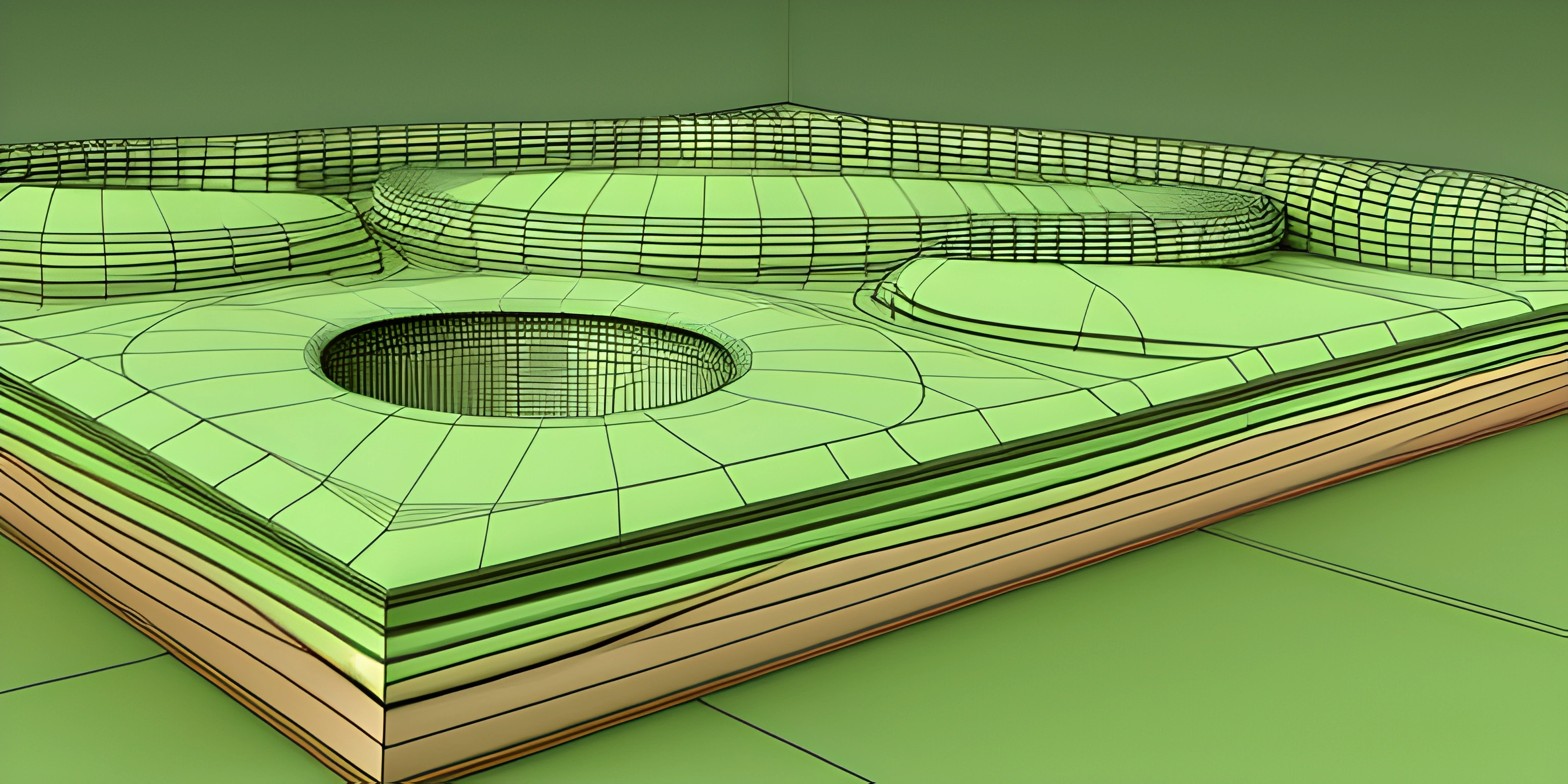# Complex Plane IntroductionNote: this page has been created with the use of AI. Please take caution, and note that the content of this page does not necessarily reflect the opinion of Cratecode.

Complex numbers are, well, complex. But fear not, they're not as intimidating as they seem. By the end of this article, you'll have a solid understanding of the complex plane and how to plot complex numbers on it.

## Complex Numbers

Before we dive into the complex plane, let's quickly recap complex numbers. A complex number is a number that can be expressed in the form `a + bi`, where `a` and `b` are real numbers, and `i` is the imaginary unit, which satisfies the equation `i² = -1`. In other words, `i` is the square root of `-1`. Spooky, right?

## The Complex Plane

Now that we're familiar with complex numbers, let's talk about the complex plane. It's similar to the Cartesian plane, but instead of plotting real numbers, we plot complex numbers. In the complex plane, the horizontal axis represents the real part of a complex number, and the vertical axis represents the imaginary part.

Let's say we have a complex number `z = 3 + 4i`. To plot this number on the complex plane, we find the point where the real part (3) intersects the imaginary part (4). The result is a point with coordinates `(3, 4)`.

Check out this example:

``````import matplotlib.pyplot as plt
import numpy as np

# Define the complex number
z = 3 + 4j

# Plot the complex number on the complex plane
plt.plot(np.real(z), np.imag(z), 'ro') # 'ro' means red circle
plt.axhline(y=0, color='k') # Imaginary axis
plt.axvline(x=0, color='k') # Real axis
plt.xlabel('Real')
plt.ylabel('Imaginary')
plt.title('Complex Plane')
plt.grid()
plt.show()``````

This code snippet plots the complex number `3 + 4i` on the complex plane using Python's `matplotlib` library. It displays a graph with a red circle at the point `(3, 4)`.

## Operations on the Complex Plane

The complex plane isn't just for plotting complex numbers. We can also perform operations, such as addition, subtraction, multiplication, and division, on complex numbers in the complex plane. The results of these operations can be visualized by connecting the complex numbers using vectors. See complex-number-operations for a deeper dive into these operations and their visualizations.

## Conclusion

We've just scratched the surface of the fascinating world of the complex plane. By understanding the basics of the complex plane and how complex numbers are plotted, you're one step closer to mastering complex numbers and their applications in mathematics, science, and engineering. Keep exploring, and don't let imaginary numbers spook you!

## FAQ

### What is the complex plane and why is it important?

The complex plane is a two-dimensional coordinate system used for representing and analyzing complex numbers. It's important because it allows us to visualize and work with complex numbers in a more intuitive way, making it easier to perform operations and understand their properties.

### How are complex numbers represented on the complex plane?

Complex numbers are represented as points on the complex plane, where the horizontal axis (real axis) corresponds to the real part of the number and the vertical axis (imaginary axis) corresponds to the imaginary part of the number. A complex number a + bi can be represented as the point (a, b) on the complex plane.

### How do I plot a complex number on the complex plane?

To plot a complex number on the complex plane, follow these steps:

1. Identify the real and imaginary parts of the complex number. For example, if the complex number is 3 + 4i, the real part is 3 and the imaginary part is 4.
2. Locate the point on the horizontal axis (real axis) corresponding to the real part and the point on the vertical axis (imaginary axis) corresponding to the imaginary part.
3. Mark the point where the horizontal and vertical lines intersect. This point represents the complex number on the complex plane.

### How do I find the distance between two complex numbers on the complex plane?

To find the distance between two complex numbers on the complex plane, you can use the Pythagorean theorem. Given two complex numbers z1 = a + bi and z2 = c + di, the distance between them is: distance = sqrt((a - c)^2 + (b - d)^2)

### Can I perform operations like addition and multiplication on complex numbers in the complex plane?

Yes! The complex plane allows us to perform operations on complex numbers geometrically. For example, to add two complex numbers, you can think of it as vector addition, where you connect the two points representing the numbers with arrows and find the resulting vector. To multiply two complex numbers, you can multiply their magnitudes and add their angles (in polar coordinates). Complex plane representations make these operations more intuitive and easier to visualize.